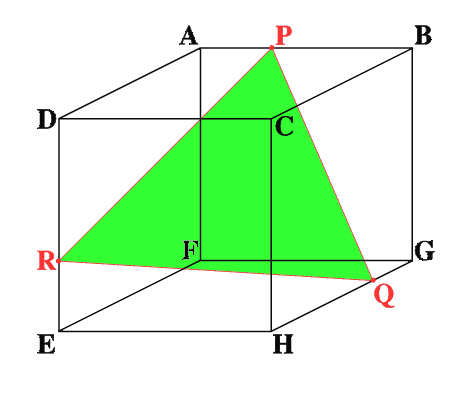#### You may also like### Kissing Triangles

Determine the total shaded area of the 'kissing triangles'.### Isosceles

Prove that a triangle with sides of length 5, 5 and 6 has the same area as a triangle with sides of length 5, 5 and 8. Find other pairs of non-congruent isosceles triangles which have equal areas.### Ratty

If you know the sizes of the angles marked with coloured dots in this diagram which angles can you find by calculation?

# Trice

##### Age 11 to 14Challenge Level

ABCDEFGH is a 3 by 3 by 3 cube. Point P is 1/3 along AB (that is AP : PB = 1 : 2),
point Q is 1/3 along GH and point R is 1/3 along ED.

To find the area of the triangle PQR, Bithian Heung's solution use Pythagoras' theorem twice and shows that triangle PQR is an equilateral triangle.

$$BQ = \sqrt {BG^2+ GQ^2} = \sqrt {3^2+ 1^2} = \sqrt {10}$$
$$PQ = \sqrt {PB^2 + BQ^2} = \sqrt {2^2 + 10} = \sqrt {14}$$

Similarly $QR = RP = \sqrt {14}$ and hence PQR is an equilateral triangle.

It is useful to remember that an equilateral triangle splits into two 30-60-90 degrees triangles. By Pythagoras theorem again the ratio of the sides is 1: $\sqrt 3$ : 2.

Hence the area of the triangle PQR is $\frac {1}{2} (7\sqrt 3)$ square units.